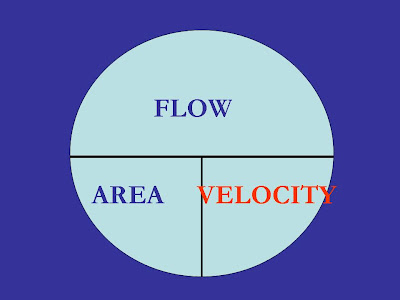# The Velocity EquationThis is your last chance to join me tomorrow in Rancho Cucamonga for the Math Review sessions. Contact the California-Nevada Section of the American Water Works Association at (909)481-7200. It’s a great way to get ready for the math portion of the State Water Treatment Operator certification exam on May 15. Because I will be in class all day, there will be no blog tomorrow — I hope to have something for you on Friday.
Today we see the third of our five key mathematical relationships, the Velocity Equation. As with our other formulas, we have three variables here, meaning we have three kinds of problems that we can solve using this one formula. But this formula has an important surprise for us, especially in treatment problems. Many apparently separate concepts are actually velocities in disguise: filtration rate, backwash rate, and surface loading rate, in particular. Most of us look at these as three different formulas that we need to memorize and learn to manipulate. But it’s actually much simpler if we recognize that each of these are really just velocities.
Velocity is another word for speed, and speed is how we usually use this formula for distribution problems: find the velocity of the water in a pipeline. To do this, we need to know the flow rate of the water in the pipe, and we also need to know the cross-sectional area of the pipe — that is, the area perpendicular to the direction of flow. In a typical pipeline problem, the area is not given to us directly; instead, we get the diameter of the pipe. From the diameter, we can find the cross-sectional area using the area of a circle, A = 0.785 d2. Note that the problem may give us the length of the pipe, too, but that is irrelevant in this calculation.
We could also find the flow rate possible in a pipeline if we know the pipe size and the water’s velocity. Similarly, we could find the size of pipe needed, if we know the maximum allowable velocity and the maximum anticipated flow rate. This last example shows up sometimes on the higher grades of Distribution exams. But it really should not: this is a problem for a design engineer! If a Distribution Operator wants to know a pipe’s size, he will either look it up or dig it up!
The Distribution application of the velocity formula will sometimes appear on the Treatment tests, but filtration rate, backwash rate, and surface overflow rate are more common. Again, each of these is a velocity, so just plug these into our formula in the velocity spot.
To find the surface overflow rate, we need to know the dimensions of our sedimentation basin. But the only ones that we will use are the length and the width — we will ignore the depth of the basin, just like we ignored the length of the pipeline above. The “Area” we need to use is the surface area, which is the length x width. This is the area that is perpendicular to the direction of flow, which is assumed to be up — toward the surface — in this calculation.
For filtration rate and backwash rate, the “Area” is the surface area of the filter. This is the length x width again, and the depth of the filter is ignored. Note that this area is perpendicular to the flow in either case! During filtration, the flow moves straight down; during backwash, the flow moves straight up.
A question that we often see in the higher grades is “How many filters are needed …” For this problem, you will normally have a maximum filtration rate (probably the regulatory maximum of 6 gallons per minute per square foot, but possibly less) and the plant’s total flow rate. Armed with this information, you can calculate the total filtration Area needed. If you know the Area of one filter, you can divide this into the total Area needed, and get the minimum number of filters required. Note that an answer of something like “6.8 filters” is nonsense, since we can’t engage “.8” filters! So always round these up to the next highest whole number — “7” in this example.
As always, keep your units straight. This is especially important here, since the velocities can be expressed in feet per second or gallons per minute per square foot.